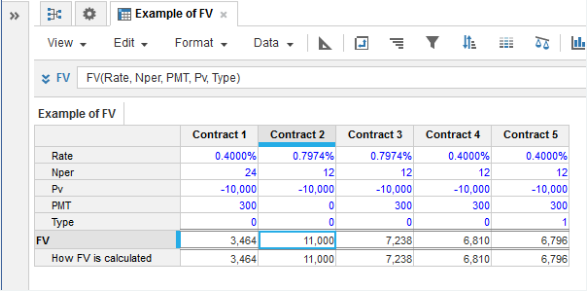1. Calculation functions
2. All Functions
3. Financial functions
4. FVCalculates the future value of an investment. This is the lump sum or closing balance you will receive back at the end of the investment.

FV Formula

FV =
IF Rate = 0 THEN
-Pv - PMT * Nper
ELSE
-Pv * (1 + Rate)Nper - PMT * (1 + Rate * Type) *((1 + Rate)Nper - 1)/ Rate

where Type=0 for payments made at the start of the period and Type=1 for payments made at the end of each period.

Syntax

FV(r, n, c, p[, t])

where:

• r: Number: Interest rate per period (Leaf-level time periods are treated as of equal length).
• n: Number: The number of periods
• c: Number: Equal payments paid out each period during the course of the investment term. Payments are recorded as a negative figure or can be zero, in which case all the interest is compounded and included in the lump sum (f) you get at the end. The payments include both an element of interest and an element of capital (optional).
• p: Number: Present value or the initial investment. Cash outflows such as investments are entered as negative numbers. If you are taking out a loan, this is a cash inflow so is entered as a positive number.
• t: Number: 0 or omitted means payments are made at the start of each period. 0 is the default if the t argument is omitted. Type=1 or non-zero means payments are made at the end of each period (optional).

Format

Input Format Output Format

Rate: Number (percentage)

Nper: Number

Pmt: Number

Pv: Number

Type: Number (binary)

Number

Arguments

The function uses the following arguments:

• r: Number: Numeric line item, property, or expression - percentage
• n: Number: Numeric line item, property, or expression
• c: Number: Numeric line item, property, or expression
• p: Number: Numeric line item, property, or expression
• t: Number: Numeric line item, property, or expression - binary

Constraints

The function has no constraints.

ExampleContract 2 involves an initial investment Pv of 10000 with a monthly interest Rate of 0.7974% per month. No payments are made during the investment (PMT = 0) so all interest is compounded and paid as a lump sum at the end. The future value FV is calculated as:

10000 * (1 + r)12
= 10000 * (1.007974)12
= 11000

Contract 3 involves the same initial investment Pv of 10000 with the same monthly interest rate Rate of 0.7974% per period (equivalent to 10% per annum compound interest) for 12 months. However, this time 12 payments of 300 (PMT) are made to the investor at the start of each month (Type). These payments are partly interest, partly capital. This means that at the end of the term, the lump sum that is returned to the investor FV is just 7238 out of an initial investment of 10000.